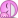# Force on a 3rd point charge due to 2 other point charges

bumdass

## Homework Statement

Two positive charges q1 = q2 = 2.0 μC are located at x = 0, y = 0.30 m and x = 0, y = -0.30 m, respectively. Third point charge Q = 4.0 μC is located at x = 0.40 m, y = 0. What is the net force ((a)magnitude and (b)direction) on charge q1 exerted by the other two charges?

## Homework Equations

F = kq1q2/r2 (where k=9*109)
a2+b2=c2
polar coordinates (r, θ) can be converted to cartesian coordinates (rcosθ, rsinθ)

## The Attempt at a Solution

I got the right answer on the first part, but not on the second part. Feel free to skip the first part and look for the next bold heading

Since q1, q2, and Q are all positively charged, they will all repel each other. Since the question only wants the net force vector acting upon q1, there are only two forces that must be looked at - the force of q2 on q1 (I'll call this Fq2q1) and the force of Q on q1 (I'll call this FQq1).

To find those forces, the first relevant equation must be used. That equation takes two charges in C, not μC, so charges must be converted (e.g. 2.0 μC = 2*10-6 C). Since both q1 and q2 have the same charge, and they are .6m apart (because .3 - (-.3) = .6; they're both sitting on the y-axis so it's easy), it's pretty straightforward to find the (magnitude of the) force here:

|Fq2q1| = k*(2*(10-6))2/(.62) = 0.1

To find the other force, similar math is used, except that since the forces aren't both on the y-axis, one must use some basic trig (relevant equation 2). Plugging in the x and y values, one gets .32 + .42 = c2, and from there one finds the distance between Q and q1 is √(.09+.16) = √(.25) = .5

|FQq1| = k*4*(10-6)*2*(10-6)/(.52) = 0.288

After computing the magnitudes, I looked for their directions so that I could have 2D vectors to describe the forces. From q2 to q1, it is obvious that the direction is 90°. From Q to q1, there is some actual math. If you plot the two points, it's easy to draw a right angle triangle where the hypotenuse is between Q and q1, and the origin touches both legs. From there, one can see the angle from Q to q1 is arctan(.3/.4) ≈ 36.8699°

Fq2q1 = (|Fq2q1|cos(90°), |Fq2q1|sin(90°))
Fq2q1 = (0, .1)

FQq1 = (0.288*cos(36.8699°), 0.288*sin(36.8699°))
FQq1 ≈ (0.2304, 0.1728)

The two vectors were found
Armed with the vectors, finding the answer to the first question was easy:
Fq2q1+FQq1 ≈ (.2304, .2728)
√(.23042+.27282) ≈ 0.3571

That answer was marked correct. But now I need the angle of Fq2q1+FQq1. To compute that, I assume all you need to do is say tan = y/x, so then the angle should be:

arctan(.2728/.2304) ≈ 49.8164

That answer was marked incorrect. I don't understand why though

Related Introductory Physics Homework Help News on Phys.org
bumdass
49.8164 degrees is in the wrong direction, so I just now tried 180-49.8164 and got the correct angleWait, this is the same exact problem I had for my physics class. Are you using MasteringPhysics as well?

I don't think I should link you to the topic with my solutions because that would constitute "giving away the answer".

bumdass
Aw man! I specifically googled the problem before posting it anywhere to see if anyone else could explain it, but I couldn't find anything :/

Nice ninja edit! I tapped on the link just in time to see your original question - yep, we had the same thing! I also have MasteringPhysics. You don't have to worry about giving away the answer, I already finished my MasteringPhysics homework this week.

Also, wow, I just now found the "Mark Solved" buttonFactChecker
•